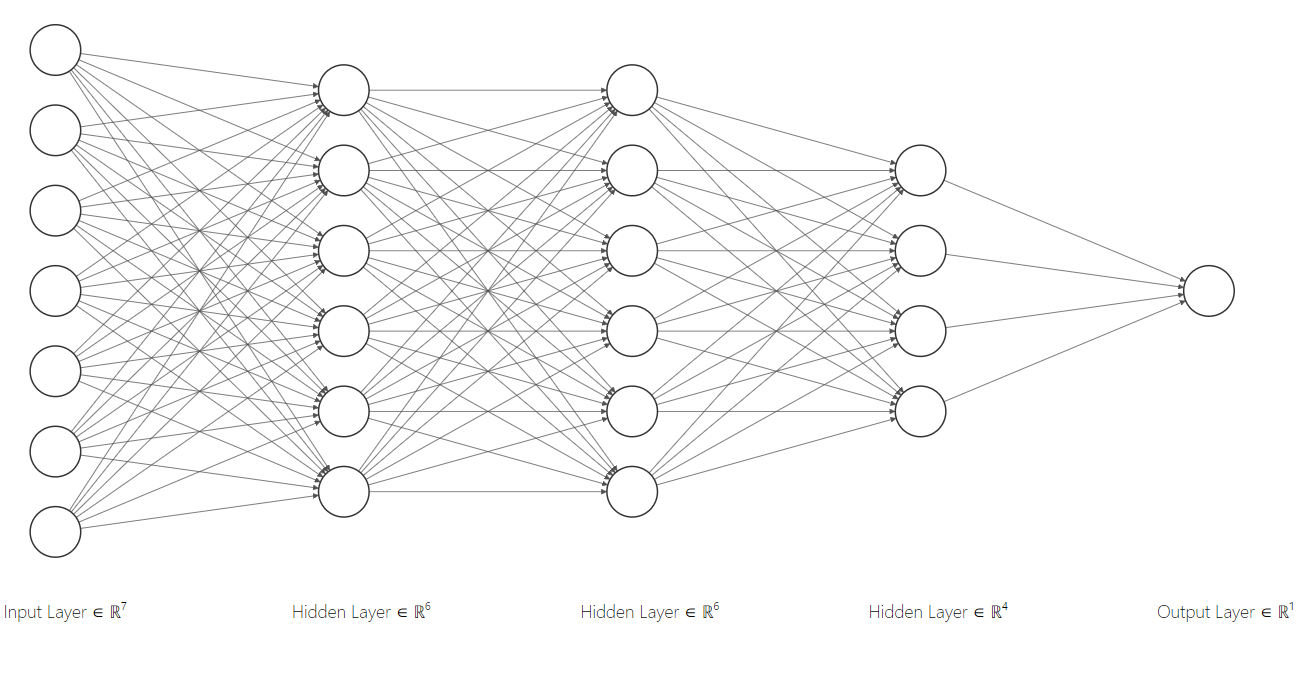# The math behind simplified Keras neural networks

What happens behind the curtain on simplified neural networks thanks to frameworks like TensorFlow and Keras

Frameworks and APIs like TensorFlow and Keras help a lot when building and running Neural Networks without having to deal with a lot of code and math. However, it's good to point out what exactly goes under the hood of a ver simplified version of a neural network that predicts house prices based on the number of flats.

## The code #

This is the neural network from the tweet. It's basically a simple neural network (1 neuron) trained in 6 lines of code (including imports).

from tensorflow import kerasmodel = keras.Sequential([keras.layers.Dense(units=1, input_shape=)])model.compile(optimizer="sgd", loss="mean_squared_error")num_of_flats = (1, 2, 3, 4, 5, 6, 7)price_of_house = (10000, 20000, 30000, 40000, 50000, 60000, 70000)model.fit(num_of_flats, price_of_house, epochs=500)

This is a pretty straight forward chunk of code for someone familiar with TF, Keras and machine learning. But it may be oversimplified for those who are just learning about machine learning and don't know what is going on under the hood.

## The magic #

The neural network build starts with the third line of code:

model = keras.Sequential([keras.layers.Dense(units=1, input_shape=)])

The Sequential model is the simplest neural network base model in Keras. Which allows us to build a model by stacking layers of nodes (neurons) on top of each other.

Each argument of the Sequential constructor is a layer of neurons; in this case, just one: a Dense layer. In dense layers (or densely-connected or fully-connected) all the neurons receive an input from all the neurons present in the previous layer. This dense layer has only one neuron (units=1) and the input is a single value (input_shape). A graphical representation of the previous sequential neural network is the following:Each neuron has an activation function which computes the value that is passed on to the neurons in the next layer. Keras layers' default is the linear activation function which multiplies each input by the weight of each neuron and returns a proportional ouput signal.

$h(x) = \sum_{i=0}^{n}(\theta_ix_i)$

Remember that we have an input with value $x_1$ (number of flats) and an initial random weight $\theta_1$, but we also have a bias with value $x_0 = 1$ and random initial weight $\theta_0$. In this simple case, the activation function of the only neuron is the actual model function:

$h(x) = \theta_0 + \theta_1x_1$

A neural network model also needs an optimizer and a loss function to evaluate its performance and adjust the weights in each iteration. That's what the following instruction is doing:

model.compile(optimizer="sgd", loss="mean_squared_error")

### Loss function #

The loss (or cost) function of a model returns a value that represent how well the model's predictions fit the training data. The target is to minimize the value that the loss function is returning by adjusting the weights accordingly. The previous model is using the Mean Squared Error (MSE) to compute this value and can be represented by the following formula:

$MSE = \frac{1}{n} \sum_{i=1}^{n}(Y_i - \hat{Y}_i)^2$

also expressed as:

$J(\theta) = \frac{1}{n} \sum_{i=1}^{n}(h_\theta(x^{(i)}) - y^{(i)})^2$

That is, if our model predicts a value of 18000 and the expected value is 20000, we have a MSE of 2000 (in one iteration).

### Optimizer #

The optimizer is the rule that our weights follow in order to adjust their values. In this case, the model is using SGD (Stochastic Gradient Descent). This function updates our weights iteratively accordingly. For every weight $\theta_j$:

$\theta_j := \theta_j + \alpha \sum_{i=1}^{n} (y^{(i)} - h_\theta(x^{(i)}))x_j^{(i)}$

$\alpha$ is the learning rate, a parameter that represents how much the weights change in each iteration

## Data #

num_of_flats = (1, 2, 3, 4, 5, 6, 7)price_of_house = (10000, 20000, 30000, 40000, 50000, 60000, 70000)

These two tuples represent our training data which will be fed to the model:

• num_of_flats: input data ($x_1$), used to feed our neuron's activation function
• price_of_house: our expected output ($y$), used to compute the cost function

## Training #

model.fit(num_of_flats, price_of_house, epochs=500)

Finally we finish by training the model by feeding it with our training data (num_of_flats, price_of_house) during 500 epochs or iterations.

The end result is a model object with trained weights so that we can make predictions with that model:

# Predict the price of a house with 10 flats>>> model.predict()99711.765625# Equivalent to: input * model.layers.get_weights() + model.layers.get_weights()

Is a library like Keras a great tool to increase your productivity as data scientist/machine learning engineer? Absolutely. Is it a great tool to learn about machine learning? Not from scratch. We can see here that the underlying mathematical theory is complex enough with a single-neuron neural network and things escalate quickly. There are other types of models apart from the Sequential, more layers than the Dense, and a ton of activation and cost functions as well as optimizers; each with a specific use-case.